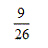Name:    Chapter 9 Post Test

Multiple Choice
Identify the choice that best completes the statement or answers the question.

1.

Find the sum.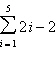a. 8 b. 12 c. 18 d. 30 e. 20

2.

Find the sum.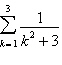a.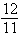b.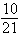c.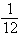d. 1 e.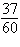3.

Use a calculator to find the sum. Round to four decimal places.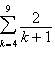a. 5.8579 b. 0.2 c. 3.8579 d. 1.4913 e. 1.6913

4.

Determine whether the sequence is arithmetic. If so, find the common difference.
5, 25, 125, 625, 3125
 a.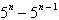b. 5 c. not arithmetic d. –5 e.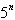5.

Write the nth term of the arithmetic sequence as a function of n.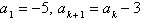a.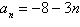b.c.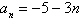d.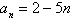e.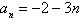6.

The first two terms of the arithmetic sequence are given. Find the indicated term.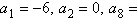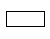a. –36 b. –42 c. 42 d. 36 e. 48

7.

Use a graphing utility to graph the first 10 terms of the sequence. (Assume that n begins with 1.) You may need to adjust the graphing window to the sizes indicated below to answer the question.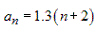a.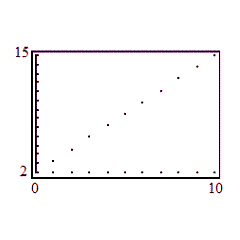d.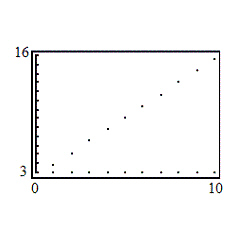b.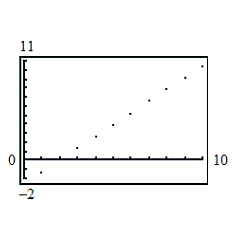e.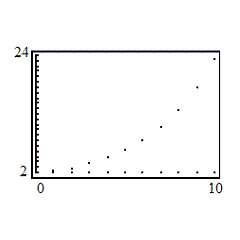c.8.

Determine whether the sequence is geometric. If so, find the common ratio.
–1, –3, –5, –7,...
 a.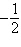b. 2 c. –2 d. not geometric e. –1

9.

Find the indicated nth term of the geometric sequence.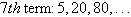a. 81,920 b. 312,500 c. 62,500 d. 29 e. 20,480

10.

Find the indicated nth term of the geometric sequence.
4th term: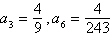a.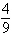b.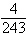c.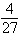d.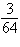e.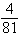11.

Find the sum using the formulas for the sums of powers of integers.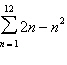a. –1482 b. –494 c. –374 d. –120 e. 78

12.

Find the specified nth term in the expansion of the binomial. (Write the expansion in descending powers of x.)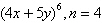a.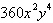b.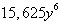c.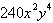d.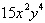e.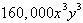13.

The probability that a basketball player will make a given free throw is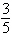. To find the probability that the player makes exactly 9 out of her next 10 free throws, evaluate the term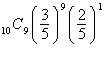in the expansion of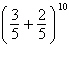. Round to four decimal places.
 a. 0.0403 b. 0.001 c. 0.1008 d. 0.0605 e. 0.0016

14.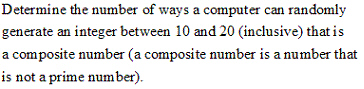a.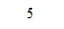b.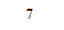c.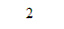d.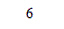e.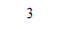15.

A combination lock will open when the right choice of three numbers (from 1 to 35) is selected. How many different lock combinations are possible?
 a. 42,875 b. 35 c. 105 d. 128,625 e. 1225

16.

Evaluate using a graphing utility: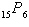a. 3,603,600 b. 5005 c. undefined d. 90 e. 360,360

17.

Find the number of distinguishable permutations of the group of letters.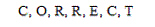a.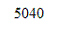b.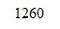c.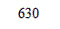d.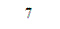e.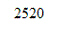18.

Nine weightlifters are competing in the dead-lift competition. In how many ways can the weightlifters finish first, second, and third (no ties)?
 a. 3 b. 27 c. 729 d. 504 e. 6

19.

Solve for n.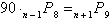a.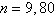b. no solution c.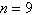d.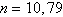e.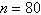20.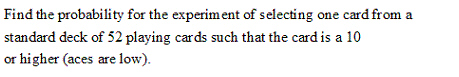a.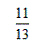b.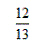c.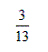d.e.